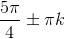## Example Questions

### Example Question #1 : How To Find The Domain Of The Tangent

What is domain of the function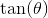from the interval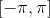?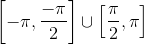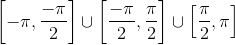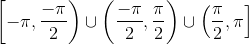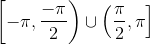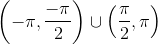Explanation:

Rewrite the tangent function in terms of cosine and sine.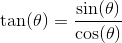Since the denominator cannot be zero, evaluate all values of theta where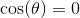on the interval.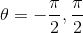These values of theta are asymptotes and will not exist on the tangent curve. They will not be included in the domain and parentheses will be used in the interval notation.

The correct solution is.

### Example Question #2 : How To Find The Domain Of The Tangent

Where does the domain NOT exist for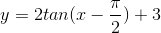?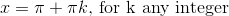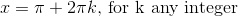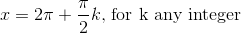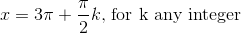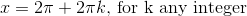Explanation:

The domain for the parent function of tangent does not exist for: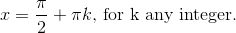The amplitude and the vertical shift will not affect the domain or the period of the graph.

The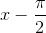tells us that the graph will shift right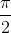units.

Therefore, the asymptotes will be located at: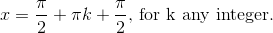The locations of the asymptotes are:### Example Question #3 : How To Find The Domain Of The Tangent

Find the domain of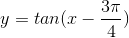.  Assumeis for all real numbers.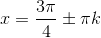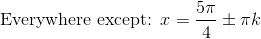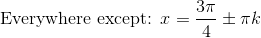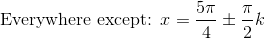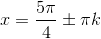Explanation:

The domain of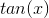does not exist at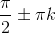, foris an integer.

The ends of every period approaches to either positive or negative infinity. Notice that for this problem, the entire graph shifts to the right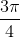units. This means that the asymptotes would also shift right by the same distance.

The asymptotes will exist at: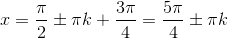Therefore, the domain ofwill exist anywhere EXCEPT: